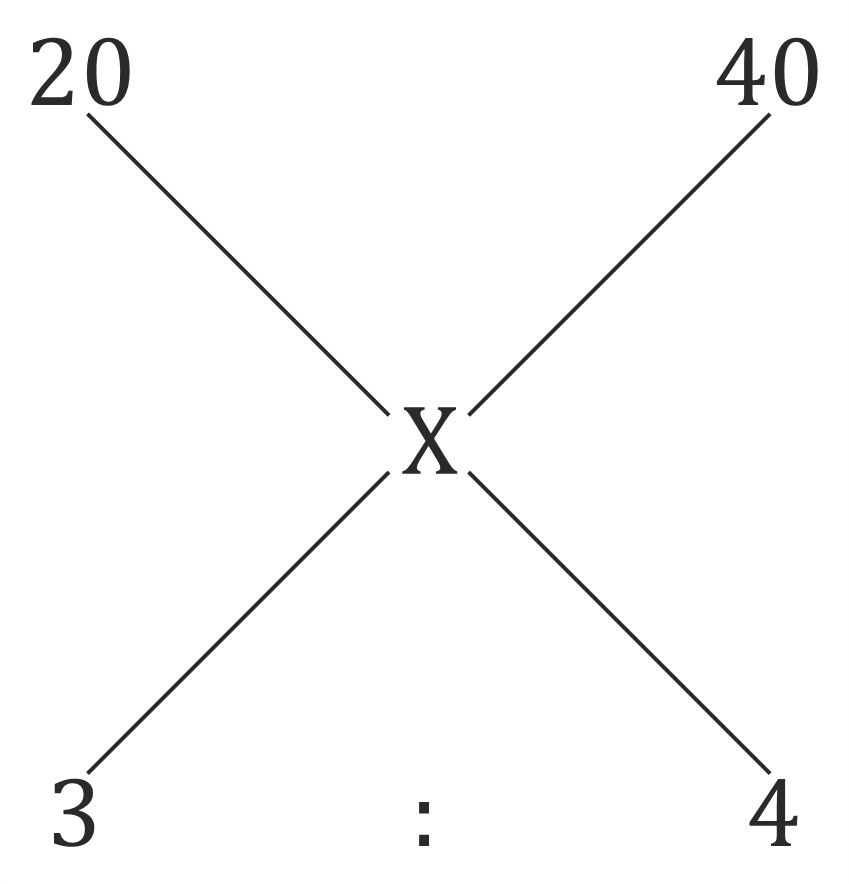We do not support landscape mode yet.
Please go back to portrait mode for the best experience
Question of The Day04-11-2019

A mixture contains two types of grains of Rs. 20 per kg and Rs. 40 per kg in the ratio 3: 4. If the seller sells the mixture at the profit of 25%, then what will be the selling price of the mixture (approximately)?

Correct Answer : b ) Rs. 40 per kg

Explanation :

Let us assume the effective cost price of the mixture after mixing both types of grains be Rs. x per kg

Using the allegation rule, we get,From the above figure we get,

$$x=\frac{20\times 3 +40\times4}{3+4}$$

$$x=\frac{220}{7}$$

Now, the seller sells the mixture at the profit of 25%

So, the selling price of the mixture will be

$$=\frac{220}{7}\times 1.25 = 39.28\approx Rs.\: 40\: per\: kg$$

Hence, (b) is the correct answer.

Basic Approach

According to the question

The mixture contains two types of grains of Rs. 20 per kg and Rs. 40 per kg in the

ratio 3: 4.

Let us assume the grain at the price of Rs. 20 per kg is 3x units

And thus, the grain at the price of Rs. 40 per kg is 4x units

Therefore, we can say that

$$The\:total\:cost\:price\:of\:new\:mixture\:obtained\:will\:be = \frac{20\times 3x+40\times 4x}{3x+4x}=Rs.\frac{220}{7}\: per\: kg$$

Therefore, the selling price of the new mixture obtained will be

$$=\frac{220}{7}+25\%\: of\: \frac{220}{7}=Rs.\frac{275}{7}=Rs.\: 39.28\approx Rs.\: 40\: per\: kg$$

Hence, (b) is the correct answer

Such type of question is asked in various exams like SSC CGL, SSC MTS, SSC CPO, SSC CHSL, RRB JE, RRB NTPC, RRB GROUP D, RRB OFFICER SCALE-I, IBPS PO, RRB Office Assistant, IBPS Clerk, RBI Assistant, IBPS RRB OFFICER SCALE 2&3, etc. Try and attempt free mock tests at PendulumEdu and upgrade your skills to excel in government exams.